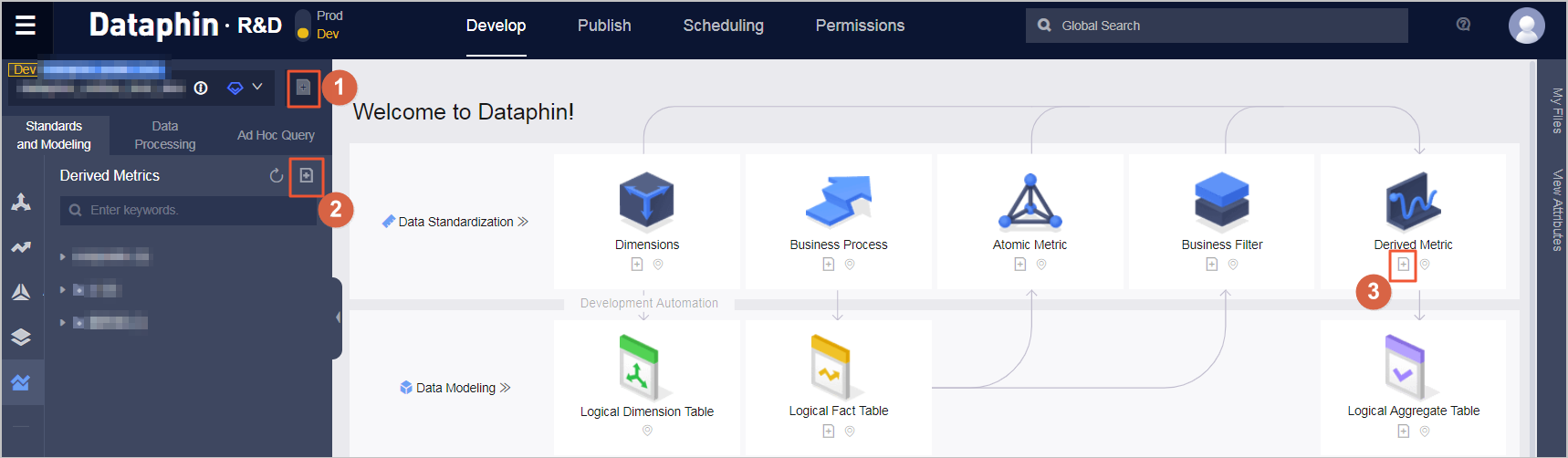This topic describes the definition of a derived metric and how to create derived metrics.

## Introduction

Derived metrics are common statistical metrics. To ensure that statistical metrics are generated in a standard and unambiguous manner, the OneData methodology categorizes derived metrics into the following four elements:

Note A derived metric consists of an atomic metric, one or more business filters, one or more statistical periods, and one or more statistic granularities.
• Atomic metric: Determines the statistical method, which is commonly known as the computing logic.
• Business filter: The business scope of statistics, which involves filtering records that meet business rules. The business filter is similar to the WHERE clause in SQL statements, excluding the WHERE keyword. However, the WHERE clause of the time interval is excluded.
• Statistical period: The time range of statistics, such as the last day and the last 30 days. The statistical period is similar to the WHERE clause of the time interval in SQL statements, excluding the WHERE keyword.
• Granularity: A statistical object or perspective that defines the fineness of data aggregation. It can be considered as a grouping condition for aggregation and similar to the object in a GROUP BY clause in SQL statements. Granularity is a combination of dimensions. For example, if a seller's turnover in a province is used as a statistical metric, the granularity is the combination of the seller and region dimensions.

Based on the preceding definitions and mechanisms, you can create multiple derived metrics without duplication in the same time. At the same time, it can ensure that the names and computing logic are clear and unique, so that business users can define these elements as needed. Derived metrics are the same level as fields and are unique within the same statistic granularity.

## Create derived metrics

1. Choose R&D > Develop > Standards and Modeling > Derived Metrics.
2. Create a derived metric. You can open the derived metric creation page by clicking one of the three icons in the following figure.3. To create a derived metric on the creation page, follow these steps: After you enter the required information as prompted, click Preview Derived Metric, and then click Submit. In the dialog box that appears, enter a description and then click OK.
4. After a derived metric is published, it is aggregated to the logical aggregate table corresponding to the statistic granularity. The system will automatically generate the logical aggregate table if it does not exist. The code of the derived metric is automatically generated by periodically task scheduling.